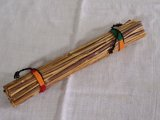The Yarrow Stalks Method

This method is fully explained in Wilhelm's translation of the I-ching, the Baynes version. Here's an abstract of the text.

The oracle is consulted with the help of yarrow stalks. Fifty stalks are used for this purpose. One is put aside and plays no further part. The remaining 49 stalks are first divided into two heaps [at random]. Thereupon one stalk is taken from the right-hand heap and put between the ring finger and the little finger of the left hand.

Then the left-hand heap is placed in the left hand, and the right hand takes from it bundles of 4, until there are 4 or fewer stalks remaining. This remainder is placed between the ring finger and the middle finger of the left hand. Next the right-hand heap is counted off by fours, and the remainder is placed between the middle finger and the forefinger of the left hand.

The sum of the stalks now between the fingers of the left hand is either 9 or 5. (The various possibilities are 1 + 4 + 4, or 1 + 3 +1, or 1 + 2 + 2, or 1 + 1 + 3; it follows that the number 5 is easier to obtain than the number 9.)The yarrow stalks pack.
You may purchase it online
from Amazon.com.
Click
here
At this first counting off of the stalks, the first stalk - held between the little finger and the ring finger - is disregarded as supernumerary, hence one reckons as follows: 9 = 8, or 5 = 4. The number 4 is regarded as a complete unit, to which the numerical value 3 is assigned. The number 8, on the other hand, is regarded as a double unit and is reckoned as having only the numerical value 2. Therefore, if at the first count 9 stalks are left over, they count as 2; if 5 are left, they count as 3. These stalks are now laid aside for the time being.

Then the remaining stalks are gathered together again and divided anew. Once more one takes a stalk from the pile on the right and places it between the ring finger and the little finger of the left hand; then one counts off the stalks as before. This time the sum of the remainders is either 8 or 4, the possible combinations being 1 + 4 + 3, or 1 + 3 + 4, or 1 + 1 + 2, or 1 + 2 + 1, so that this time the chances of obtaining 8 or 4 are equal. The 8 counts as 2, the 4 counts as 3.

The procedure is carried out a third time with the remaining stalks, and again the sum of the remainders is 8 or 4.

Now, from the numerical values assigned to each of the three composite remainders, a line is formed.

If the sum is 5 (= 4, value 3) + 4 (value 3) + 4 (value 3), the resulting numerical value is 9, the so-called old yang. This becomes a positive line that moves and must therefore be taken into account in the interpretation of the individual lines. It is designated by the symbol O or O.

If the sum of the composite remainders is 9 (= 8, value 2) + 8 (value 2) + 8 (value 2), the final value is 6, the so-called old yin. This becomes a negative line that moves and is therefore to be taken into account in the interpretation of the individual lines. It is designated by the symbol - X - or X.

If the sum is

9 (2) + 8 (2) + 4 (3)
or 5 (3) + 8 (2) + 8 (2)    = 7
or 9 (2) + 4 (3) + 8(2)

the value 7 results, the so-called young yang. This becomes a positive line that is at rest and therefore not taken into account in the interpretation of the individual lines. It is designated by the symbol ____ .

If the sum is

9 (2) + 4 (3) + 4 (3)
or 5 (3) + 4 (3) + 8 (2)   = 8
or 5 (3) +8 (2) +4 (3)

the value 8 results, the so-called young yin. This becomes a negative line that is at rest and therefore not taken into account in the interpretation of the individual lines. It is designated by the symbol
__  __ .

This procedure is repeated six times, and thus a hexagram of six stages is built up.Consult the I-ching oracle  by clicking here. Or ask for online assistance here .

<= Back to the Oracle page

 Home© 1999-2019, Way of Perfect Emptiness. All rights reserved. Updated:   25 septembrie 2019